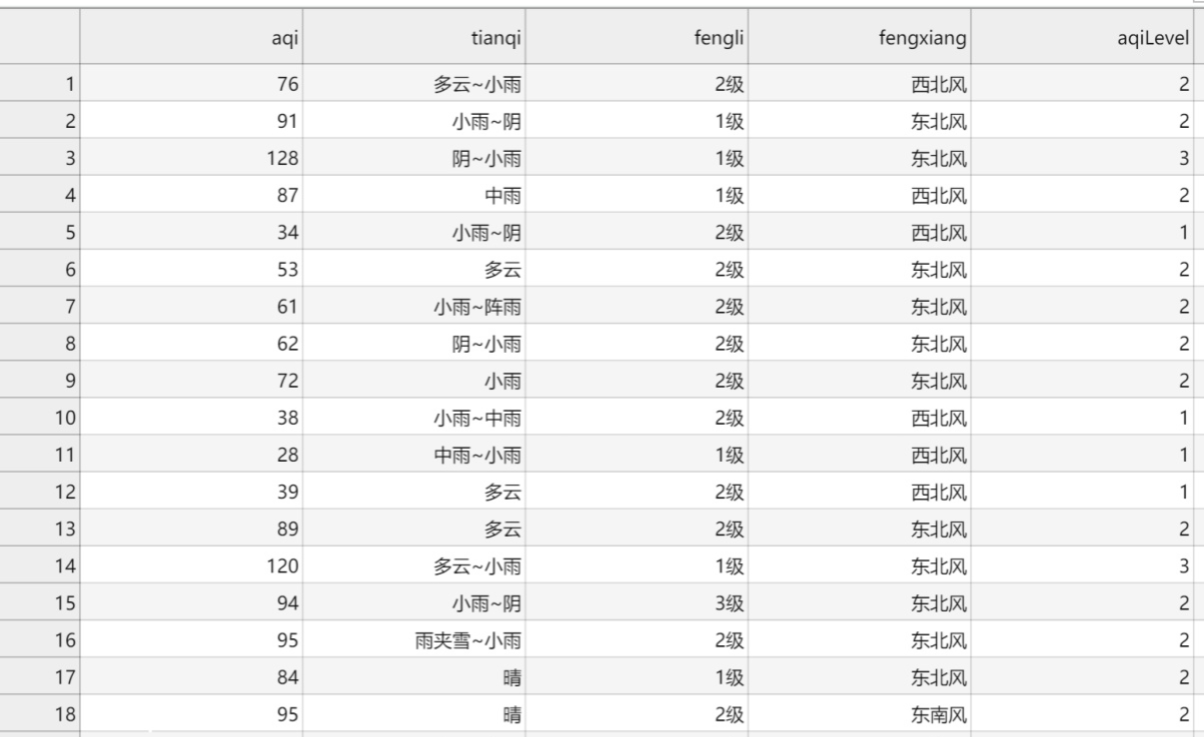• 写文章
• 提问

# python爬虫可以用来看天气预报吗？1 构造URL列表

```# 构造2019全年的月份列表
months = []
for year in (2019,):
for month in range(12):
months.append("%d%02d"%(year, month+1))
todo_urls = [
"http://tianqi.2345.com/t/wea_history/js/"+month+"/58457_"+month+".js"
for month in months
]```

2 批量下载数据

```import requests
datas = []
for url in todo_urls:
if r.status_code!=200:
raise Exception()
# 去除javascript前后的字符串，得到一个js格式的JSON
data = r.text.lstrip("var weather_str=").rstrip(";")
datas.append(data)```

3 解析数据

```# 解析所有月份的数据
all_datas = []

for data in datas:
tqInfos = demjson.decode(data)["tqInfo"]
all_datas.extend([x for x in tqInfos if len(x)>0])```

4 将结果导入 csv 文件

```import csv
with open('./hangzhou_tianqi_2019.csv', 'w', newline='', encoding='utf-8') as csv_file:
writer = csv.writer(csv_file)
columns = list(all_datas.keys())
writer.writerow(columns)

for data in all_datas:
writer.writerow([data[column] for column in columns])```

5 结果展示